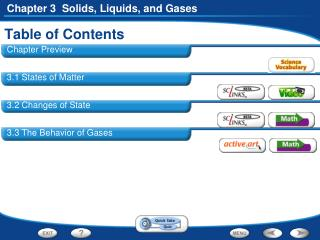DownloadDownload PresentationDownload Presentation- - - - - - - - - - - - - - - - - - - - - - - - - - - E N D - - - - - - - - - - - - - - - - - - - - - - - - - - -
##### Presentation Transcript

1. Table of Contents • Chapter Preview • 3.1 States of Matter • 3.2 Changes of State • 3.3 The Behavior of Gases

2. Section 3:The Behavior of Gases • What types of measurements are useful when working with gases? • When working with a gas, it is helpful to know its volume, temperature, and pressure.

3. Section 3:The Behavior of Gases • How are the volume, temperature, and pressure of a gas related?

4. A Change in Pressure • The pressure of the gas is the force of its outward push divided by the area of the walls of the container. • A punctured basketball deflates as gas particles begin to escape.

5. Temperature and Volume • When the temperature of a gas increases at constant pressure, its volume increases.

6. Pressure and Temperature • When a gas is heated, the particles move faster and collide more often with each other and with the walls of their container. The pressure of the gas increases.

7. Charles’ Law • Charles’ Law states that at constant pressure, the volume of the gas increases as its temperature increases. Likewise, as the volume of the gas decreases its temperature decreases.

8. Charles’ Law • The graph is directly proportional, that is, when a graph of two variables is a straight line passing through the origin.

9. Charles’s Law Video • Optional video for extra work htp://teachertube.com/viewVideo.php?video_id=92871&title=Gases_05___Charles_Law http://www.youtube.com/watch?v=IkRIKGN3i0k

10. Pressure and Volume • As weights are added, the gas particles occupy a smaller volume. The pressure increases.

11. Boyle’s Law • Boyle’s Law states that at constant temperature, the volume of a gas increases as its pressure decreases

12. Boyle’s Law • A graph of Boyle’s Law will be inversely proportional, which is when the product of two variables is a constant.

13. Gas Laws Activity • Click the Active Art button to open a browser window and access Active Art about gas laws. http://preparatorychemistry.com/Bishop_Boyles_Law_Flash1.htm

14. Graphing Gas Behavior In an experiment, the temperature of a gas at a constant volume was varied. Gas pressure (in kilopascals) was measured after each 5-kelvin change in temperature. The data from the experiment are shown in the table.

15. Graphing Gas Behavior Graphing Use the data to make a line graph. Plot temperature on the horizontal axis with a scale from 270 K to 300 K. Plot pressure on the vertical axis with a scale from 0 kPa to 25 kPa. (1 kPa = 1,000 Pa)

16. Graphing Gas Behavior Interpreting Data What was the manipulated variable in this experiment? Changing the temperature of a gas at constant volume changes its pressure in a similar way.

17. Graphing Gas Behavior Interpreting Data What kind of trend do the data show? Pressure increases with temperature.

18. Graphing Gas Behavior Drawing Conclusions What happens to the pressure of a gas when temperature is increased at constant volume? Gas pressure increases.

19. End of Section:The Behavior of Gases